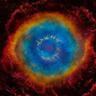Magicode# 04.05 Histograms, Binnings, and DensityA simple histogram can be a great first step in understanding a dataset. Earlier, we saw a preview of Matplotlib's histogram function (see Comparisons, Masks, and Boolean Logic), which creates a basic histogram in one line, once the normal boiler-plate imports are done:%matplotlib inline
import numpy as np
import matplotlib.pyplot as plt
plt.style.use('seaborn-white')

data = np.random.randn(1000)plt.hist(data);The hist() function has many options to tune both the calculation and the display; here's an example of a more customized histogram:plt.hist(data, bins=30, normed=True, alpha=0.5,
histtype='stepfilled', color='steelblue',
edgecolor='none');The plt.hist docstring has more information on other customization options available. I find this combination of histtype='stepfilled' along with some transparency alpha to be very useful when comparing histograms of several distributions:x1 = np.random.normal(0, 0.8, 1000)
x2 = np.random.normal(-2, 1, 1000)
x3 = np.random.normal(3, 2, 1000)

kwargs = dict(histtype='stepfilled', alpha=0.3, normed=True, bins=40)

plt.hist(x1, **kwargs)
plt.hist(x2, **kwargs)
plt.hist(x3, **kwargs);If you would like to simply compute the histogram (that is, count the number of points in a given bin) and not display it, the np.histogram() function is available:counts, bin_edges = np.histogram(data, bins=5)
print(counts)
[ 12 190 468 301 29]

### Two-Dimensional Histograms and Binnings

Just as we create histograms in one dimension by dividing the number-line into bins, we can also create histograms in two-dimensions by dividing points among two-dimensional bins. We'll take a brief look at several ways to do this here. We'll start by defining some data—an x and y array drawn from a multivariate Gaussian distribution:mean = [0, 0]
cov = [[1, 1], [1, 2]]
x, y = np.random.multivariate_normal(mean, cov, 10000).T

#### plt.hist2d: Two-dimensional histogram

One straightforward way to plot a two-dimensional histogram is to use Matplotlib's plt.hist2d function:plt.hist2d(x, y, bins=30, cmap='Blues')
cb = plt.colorbar()
cb.set_label('counts in bin')Just as with plt.hist, plt.hist2d has a number of extra options to fine-tune the plot and the binning, which are nicely outlined in the function docstring. Further, just as plt.hist has a counterpart in np.histogram, plt.hist2d has a counterpart in np.histogram2d, which can be used as follows:counts, xedges, yedges = np.histogram2d(x, y, bins=30)
For the generalization of this histogram binning in dimensions higher than two, see the np.histogramdd function.

#### plt.hexbin: Hexagonal binnings

The two-dimensional histogram creates a tesselation of squares across the axes. Another natural shape for such a tesselation is the regular hexagon. For this purpose, Matplotlib provides the plt.hexbin routine, which will represents a two-dimensional dataset binned within a grid of hexagons:plt.hexbin(x, y, gridsize=30, cmap='Blues')
cb = plt.colorbar(label='count in bin')plt.hexbin has a number of interesting options, including the ability to specify weights for each point, and to change the output in each bin to any NumPy aggregate (mean of weights, standard deviation of weights, etc.).

#### Kernel density estimation

Another common method of evaluating densities in multiple dimensions is kernel density estimation (KDE). This will be discussed more fully in In-Depth: Kernel Density Estimation, but for now we'll simply mention that KDE can be thought of as a way to "smear out" the points in space and add up the result to obtain a smooth function. One extremely quick and simple KDE implementation exists in the scipy.stats package. Here is a quick example of using the KDE on this data:from scipy.stats import gaussian_kde

# fit an array of size [Ndim, Nsamples]
data = np.vstack([x, y])
kde = gaussian_kde(data)

# evaluate on a regular grid
xgrid = np.linspace(-3.5, 3.5, 40)
ygrid = np.linspace(-6, 6, 40)
Xgrid, Ygrid = np.meshgrid(xgrid, ygrid)
Z = kde.evaluate(np.vstack([Xgrid.ravel(), Ygrid.ravel()]))

# Plot the result as an image
plt.imshow(Z.reshape(Xgrid.shape),
origin='lower', aspect='auto',
extent=[-3.5, 3.5, -6, 6],
cmap='Blues')
cb = plt.colorbar()
cb.set_label("density")